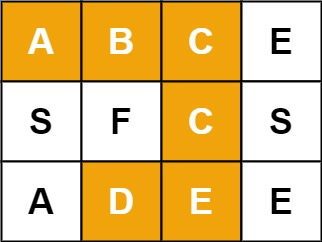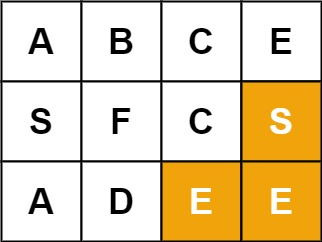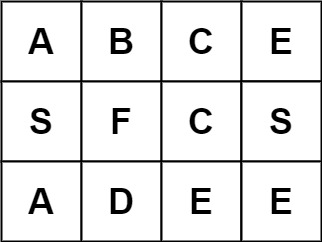# 79. Word Search

Medium
Given an `m x n` grid of characters `board` and a string `word`, return `true` if `word` exists in the grid.
The word can be constructed from letters of sequentially adjacent cells, where adjacent cells are horizontally or vertically neighboring. The same letter cell may not be used more than once.
Example 1:Input: board = [["A","B","C","E"],["S","F","C","S"],["A","D","E","E"]], word = "ABCCED"
Output:
true
Example 2:Input: board = [["A","B","C","E"],["S","F","C","S"],["A","D","E","E"]], word = "SEE"
Output:
true
Example 3:Input: board = [["A","B","C","E"],["S","F","C","S"],["A","D","E","E"]], word = "ABCB"
Output:
false
Constraints:
• `m == board.length`
• `n = board[i].length`
• `1 <= m, n <= 6`
• `1 <= word.length <= 15`
• `board` and `word` consists of only lowercase and uppercase English letters.
Follow up: Could you use search pruning to make your solution faster with a larger `board`?

### 解題

Runtime: 67 ms, faster than 95.23%
Memory Usage: 1.9 MB, less than 92.11%
func exist(board [][]byte, word string) bool {
for i := range board {
for j := range board {
if dfs(board, word, i, j) { return true }
}
}
return false
}
func dfs(board [][]byte, word string, i int, j int) bool {
if len(word) == 0 {
return true
}
if i < 0 || i > len(board) - 1 || j < 0 || j > len(board) - 1 {
return false
}
if board[i][j] != word {
return false
}
temp := board[i][j]
board[i][j] = '#'
if dfs(board, word[1:], i + 1, j) || dfs(board, word[1:], i, j + 1) || dfs(board, word[1:], i - 1, j) || dfs(board, word[1:], i, j - 1) {
return true
}
board[i][j] = temp
return false
}